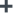# Fibonacci ratio of 61.8% suggests buying opportunity in ITC

ITC traded higher in the previous week and the uptrend is likely to continue; Rs 160 should be considered the stoploss level on a closing basis, says Shabbir Kayyumi of Narnolia Financial Advisors. .
Oct 26, 2020 / 01:28 PM ISTlive
• bselive
• nselive
Volume
Todays L/HMore

What are Fibonacci Ratios?

Fibonacci retracements are popular among technical traders. They are based on the key numbers identified by mathematician Leonardo Fibonacci in the 13th century. Fibonacci's sequence of numbers is not as important as the mathematical relationships, expressed as ratios, between the numbers in the series.

The Fibonacci sequence is as follows:

0, 1, 1, 2, 3, 5, 8, 13, 21, 34, 55, 89, 144, 233, 377, 610…

Each number in the series is the addition of the previous two numbers as shown below.
233 = 144 + 89
144 = 89 + 55

89 = 55 +34

There are some interesting properties of the Fibonacci series. Further into the ratio properties, one can find remarkable consistency when a number is in the Fibonacci series is divided by its immediate succeeding number.

For example:
89/144 = 0.618
144/233 = 0.618

377/610 = 0.618

The ratio 0.618 when expressed in percentage is 61.8 percent.

In technical analysis, a Fibonacci retracement is created by taking two swing points or two pivot points, on a stock chart and dividing the vertical distance by the key Fibonacci ratios of 23.6 percent, 38.2 percent, 50 percent, 61.8 percent, and 100 percent.

Fibonacci retracements are popular tools that traders use to draw support lines, identify resistance levels, place stop-loss orders and set target prices. Fibonacci retracements suffer from the same drawbacks as other universal trading tools, so they are best used in conjunction with other indicators.

Generally, prices retrace towards the important ratio of 61.8 percent as a part of correction, from there chances of taking support and showing reversal move is highly possible.

In the case of ITC, prices touched 61.8 percent ratio recently, took support and managed to trade higher in the previous week. There are chances that original trend will continue as long as ITC trades above the 164-mark. At the same time, prices managed to close above the high of the last seven trading sessions, a sign of trend reversal and breakout too. Moreover, this stock has closed above its short-term moving average 20 DMA, standing at around 169-mark, indicating that the correction is complete and the original up-trend will resume.Figure.1. Fibonacci retracement (61.8 percent) and Buy signal on ITC

Profit booking

Looking at Fibonacci retracement levels and anticipating conclusion of correction leg, one can initiate long position for higher targets of 23.6 percent retracements. However, moving further Rs 205 will attract more profit-booking. So one can consider booking profit near Rs 192 and higher side towards Rs 205 mark.

Stop Loss

The entire bullish view negates on a breach of the immediate swing low and in for ITC, we will consider Rs 160 as a stoploss level on a closing basis.

Conclusion

We recommend buying ITC at around Rs 170 with a stop loss of Rs 160 for higher targets of Rs 192 as indicated in the above chart.